﻿ Estimation Comparison of Multidimensional Reliability Coefficients Measurement of Senior High School Students’ Affection towards Mathematics

### Estimation Comparison of Multidimensional Reliability Coefficients Measurement of Senior High School...

Wardani Rahayu, Dogol Harjono

American Journal of Educational Research

## Estimation Comparison of Multidimensional Reliability Coefficients Measurement of Senior High School Students’ Affection towards Mathematics

Wardani Rahayu1,, Dogol Harjono2

1Mathematics and Science Department, Universitas Negeri Jakarta, Indonesia

2Research and Educational Evaluation Program Study, Universitas Negeri Jakarta, Indonesia, Kampus UNJ, Jl. Rawamangun Muka, Rawamangun, Jakarta

### Abstract

The purposes of this study were to develop instrument of high school students’ affection towards mathematics. It also compared estimation of multidimensional reliability coefficients of the instrument, i.e. McDonald reliability and Maximum reliability coefficient. The research used multi-stage random sampling technique. Conceptually, construct of the affection instrument consists of 5 dimensions, namely: attitude, interest, self-concept, values, and morals. Inter-rater reliability coefficient which were obtained for the five dimensions move from 0.73 to 0.80 and produce 86 selected items. These results were achieved from the items which have been selected through expert study and panelists’ validation. Empirically, first and second trials (try out) testing which used confirmatory factor analysis (CFA) with Maximum Likelihood method (ML) recieved loading factor value above 0,3. In the first and second trials, the multidimensional reliability coefficient calculation showed that the value of McDonald and Maximum reliability coefficients were more than 0.9, which means that the level of reliability of the affection instrument towards mathematics of high school students classified in a very high level. Hypothesis testing of McDonald and Maximum reliability coefficient used t-paired test on bootstrapping methods samples. The result is McDonald reliability coefficient is lower than Maximum reliability coefficient.

• Wardani Rahayu, Dogol Harjono. Estimation Comparison of Multidimensional Reliability Coefficients Measurement of Senior High School Students’ Affection towards Mathematics. American Journal of Educational Research. Vol. 3, No. 11, 2015, pp 1444-1449. http://pubs.sciepub.com/education/3/11/15
• Rahayu, Wardani, and Dogol Harjono. "Estimation Comparison of Multidimensional Reliability Coefficients Measurement of Senior High School Students’ Affection towards Mathematics." American Journal of Educational Research 3.11 (2015): 1444-1449.
• Rahayu, W. , & Harjono, D. (2015). Estimation Comparison of Multidimensional Reliability Coefficients Measurement of Senior High School Students’ Affection towards Mathematics. American Journal of Educational Research, 3(11), 1444-1449.
• Rahayu, Wardani, and Dogol Harjono. "Estimation Comparison of Multidimensional Reliability Coefficients Measurement of Senior High School Students’ Affection towards Mathematics." American Journal of Educational Research 3, no. 11 (2015): 1444-1449.

 Import into BibTeX Import into EndNote Import into RefMan Import into RefWorks

1
Prev Next

### 1. Introduction

A success mathematics lesson in cognitive and psychomotor aspects is affected by the affective condition of students. Well motivated and positive behavior students’ affection towards mathematics will make them enjoy the lesson, so that they can get optimal achievement. Teacher has to concern about affective characteristics of students in designing learning program and activities in order to reach an optimal learning outcome. There are 5 characteristics to evaluate students’ affective which include observation sheets, namely: (1) behavior, (2) interest, (3) self-concept, (4) value, and (5) moral.

Affection is a general term that refers to a particular mood and emotion (Schunck, Pintrich, and Meece, 2008: 375). The aims of affection are: (1) entertainments: fun experiences, passions, boredom prevention, stress control, (2) serenity: relax and comfortable senses, overstress avoidence, (3) happiness: joyfulness, satisfaction, emotional pressure prevention, (4) physical senses: enjoyment that requires physical sensation, movement, or contact, avoid unpleasant things, and (5) physical benefits: feeling healthy, energetic, fatigue or sickness avoidence. The impact of affection is usually generalized as behavior controlling. The purpose of controlling has been related to positive attitude towards learning assignments, as well as believes in values. 

Affective domain which is exposed by Krathwhol, Bloom, and Masia quoted in Smith (2009) covers conscious behaviors, interest, concerns, responsibility, listening ability, responsiveness, interaction, and an ability to demonstrate a proper attitude in a test or lesson situation.  Affective domain relates to emotion, attitude, appreciations, and some values such as comfort, preserve, respect, and support. According to Bloom, characteristic of affection is a combination of concerns, attitudes and self-esteem. Such affection can be related to lesson, school and learners’ academic self-concept. 

Affection domain is classified into: (a) receiving, (b) responding, (c) rewarding (valuing), organizing, and (e) charactering.  The five categories of affection has an internaly continuous range which covers interest, attitude, value, appreciation, and adjustment. Such elements are intersecting to each other in a view of an individual process.

The affective aspects in mathematics education covers: belief, attitude, and emotion. The idea of belief and attitude towards mathematics is connected to teacher’s and students’ value. Belief and attitude in math education give more concern to a fact that there is an affection behavior aspect, named as value. Behavior aspect becomes a significant focus of study on attitude, belief, and value development. 

Students’ success in learning process can’t always be measured with a test tool, since there are still many aspects of students’ ability which are difficult to be measured quantitatively and objectively. For example, mathematics affective aspects cover attitude, interest, diligence, honesty, responsibility, tolerance, solidarity, belief or optimism, and so forth. Therefore, an appropriate and qualified evaluation tool is necessary in order to measure such aspects. One of the measurement tools which can be used to measure students’ affective aspects on mathematics lessons is a non-test instrument.

The developments of Senior High School students’ mathematical affection instruments are usually use a single dimension (uni dimentional) instrument. This measurement conceptually formulated to a type of attitude or factor of interest which is measured by one measurement instrument. However, many researchs show that such single dimension assumption is hard to be reached due to some appearence of some new factors which are also measured in one instrument. In other words, mathematical affection instrument has a multi dimensional characteristic. 

Some studies were done in order to measure dimensionality. For example, a research was done by Widhiarso (2009).  The research discusses about estimation of alpha coefficient which has a single dimension. Alpha coefficient resulted in a high reliability coefficient on all items. In this case, estimation of alpha coefficient goes from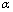= 0,836 up to= 0,961. In the case of multi dimensional data with small number of items (less than 15), alpha coefficient produced a low coefficient reliability. In contrary, data with big number of items (more than 15) resulted in a high reliability alpha coefficient. The result of the research shows that alpha coefficient is less sensitive towards data dimensionality when the numbers of items are more than 15. It can be seen from a quite high reliability value (more than 0.7) on various numbers of dimensions.

Most researchers use reliability coefficient monotonously without considering assumption based coefficient. It is expected that the reliability study is not only fixed with one coefficient only. It also involves reliability which is likely to draw more optimal results. Most researchers only focus on using Alpha Cronbach coefficient to estimate reliability without firstly comprehends the assumptions that underlie such coefficient. Many researchers do not realize that alpha coefficient requires particular assumption to be filled. If this assumption is not filled, then the reliability coefficient which is resulted from such assumption is the lowest estimation limit (underestimate). 

One assumption that estimates alpha coefficient is single dimensionality (uni dimentional) data. If alpha coefficient is applied to multi dimensional measurement, it will produce an underestimate result. Therefore, a research which is going to identify multi dimensional measurement reliability should use reliability coefficient which can accommodate multidimensional characteristics. 

Reliability coefficient for Structural Equation Model (SEM) based- multi dimensional measurement is started with confirmatory factors analysis, such as maximum reliability coefficient, McDonald’s construct reliability coefficient and Raykov’s composite reliability coefficient. A question arises from this fact is which reliability coefficient is accurate for multi dimensional measurement model. This research will expose some reliability coefficients which can be applied on multi dimensional measurement model, namely McDonald’s constructive reliability coefficient and maximum reliability coefficient, and also compares the estimation accuracy of each coefficient.

### 2. Method

This research applied survey method by giving questionnaires to respondents. Survey was used for data collection which was intended to reveal facts based on the indications that was recieved from correspondents’ answers. The questionnaires were psychology attribute which were related to math. Likert scale was used with five categories of answers, namely strongly agree, agree, neutral, disagree, and strongly disagree. The method which was oriented on stimulus or responses from respondents used to determine position of continuum. The resulted scores will be the score of the agreed item.

The scores, which were obtained from trial, were analyzed in order to emphasize construct validation as well as their reliability coefficient by using confirmatory factor analysis with ML (Maximum Likelihood) method. Hypothesis testing of multi dimensional reliability coefficient differences between McDonald and Maximal methods were performed through t-paired test on bootstrapping method sample.

#### Table 1. Research Design on Bootstrapping Data

Affection towards math is a psychology response in form of somebody’s feeling or emotion towards math. Positive statement can be expressed in form of respect, enjoyment, or sympathy. Negative statement can be expressed in form of fear, refusal, hate or dislike. Dimension and indicator of affection construction towards math which is going to be measured is received from theoretical study. Dimension and indicator from affection construction towards math can be seen on Table 2 below.

### 3. Research Findings

Based on analysis of experts, they give relatively similar evaluation in regards to construct the affection performance test towards mathematics for senior high school students. Generally, the arranged indicators have been considered as a representation of dimension of affections’ construct towards math. The arranged indicators are the representations of defined construct. In other words, the item construction is in accordance with the indicator.

The experts give some inputs to the items statements which are arranged based on each indicator. There are some items of statements which are overlapping, for example, the item should be included in behavioral dimension, yet it still appears in dimension of interest or even in other dimensions. Therefore, the experts need to give suggestion to make changes to the construction of items statements so it will adjust to indicator and dimension. It is done by picking such item to be included into the most appropriate, dominant, and representative dimension to measure the indicator.

The result of experts’ analysis towards non test instrument, which is the guidelines for filling out the questionnaire, shows that generally the non-test instruments are sufficient when are noticed from grammar and writing usage. Experts considered that language that was used in non test instruments is communicative. However, there are some sentences which are suggested to be changed because they are difficult to be understood, confusing and they may cause misinterpretation.

Besides that, the experts have to give some inputs for refinement, such as: by avoiding continous words from left to the right, like “often”, “always”, “very”; avoid items which can be misinterpreted as facts when they are not; avoid items which can be interpreted in many different ways, it is usually found in items that contain conjunction of “and”, ”but”; avoid items which answers will likely to be similar from every respondent or items which will not be chosen by any respondent; to draft the items with a simple, clear, and direct language; make the items shorts, not more than 20 words; one item only contains one idea/main idea; avoid double negative statement; and avoid items which may create ambiguity for respondents.

In accordance to experts’ suggestions which concerned on construct validity and readable factor of items in statements, grammar use, and writing. As a result, there are 23 items which should be canceled and excluded from the analysis. The items are actually already included in other items so that they are overlapped. Therefore, there are 86 items which have fulfilled requirements on affection measurement performance test towards math for senior high school students. This measurement is going to be used for validity and reliability test analysis.

The result of improvement and refinement on non test instrument was then rechecked by 20 panelists on a rational test. The purpose of this test was to determine fitness and reliabilities of the items statements between raters. CVR (Content Validity Ratio) from Lawshe was applied for fitness test of items statements which involved 20 panelists (rater). It can be seen that all items statements on each dimension already matched with and adjusted to its’ dimension construction.

It can be seen from the calculated CVR scores which exceeds CVR tables’ critical scores of on 5% significance test with 20 raters, which is 0.42. Thus, all items can be considered as appropriate and fit to be used to measure senior high school students’ affective construction of towards math. Hoyt formula was used to calculate the measurement of construct reliability from the panelists towards the affection with non test instruments. The result of coefficient reliability from inter rater reliability calculation from panelists for each dimension moves from 0.73 to 0.80. These scores are classified as a high level reliability so that the affection instrument towards math for Senior High School students are considered as a reliable instrument.

The empirical test calculation shows that there are three items of statements which are not valid and need to be dropped because they have rcalculation value which are less than 0.2. The reliability test by using coefficient reliability formula from Alpha Cronbach is the next step to be done for each dimension.

The result shows that reliability coefficient scores for each dimension have an alpha Cronbach score which is moved from 0.712 to 0.881. Based on the criteria that expressed by Naga (2012), it can be stated that measurement tool which is used in this research is appropriate and reliable.  Since this affection instrument is multi dimensional, the measurement of coefficient reliability is also performed with multi dimensional coefficient reliability formula. One of the formula is stratified with alpha coefficient. The result of the calculation produces a score of stratified alpha coefficient, that is αs = 0,794. Such reliability coefficient score is considered as a high reliability. As a result, the affection instrument towards math for Senior High School students which has multidimensional characteristic can be used as a reliable measurement tool.

Composite score is generated from the addition of each items that suit to its’ indicator. After that, by using confirmatory factor analysis, such indicators are confirmed to be known if they have been in accordance to its' basic dimension of affection towards math. Goodness of fit in SEM can’t be directly performed like other multivariate techniques. SEM does not have best statistic test which can explain the strength of model prediction. Therefore, some measurements of goodness of fit which can be used supportively need to be developed.

This test is conducted in order to evaluate the goodness of fit (GOF) between data and model. Generally, model fitness test for involves structural and integrated model are divided into three groups of test: absolute fit measures, incremental fit measures, and parsimonious fit measures.

#### Table 3. Total Scores of Model Goodness of Fit Measurement on 1st Trial

Table 2 shows that there are 2 GOF measurements that shows a not really good compatibility result, which are Chi Square and RMR. There are 2 GOF measurements which are marginal fit, GFI and AGFI, and 10 GOF measurements which show good fit. The result indicates that even though there are some GOF measurements which show a not really good fit, most of GOF measurements shows a good fit. Therefore, it can be concluded that the most model is good fit.

Evaluation of measurement model fitness is done to each construct by looking at the validity and evaluation of its construct reliability. Measurement model testing is done through convergent validity and reliability testing. Convergent validity shows that measurement indicators (manifest variables) from a latent construct should have high correlation. Reliability testing is needed to know the instrument accuracy, consistency, and precision in measuring construct.

Convergent validity can be seen from loading factor value of indicators from each dimension with an acceptable loading factor value above 0.30. The loading factor value and t-value of each indicator in every dimension can be seen as the following figure.

Figure 1 shows that all indicators are significant because the loading factor value is > 0.3. Therefore, it can be stated that construct indicators for each dimensions can explain latent construct very well. The result of validity test by concerning loading factor is also relevant with the t-test. This shows tcalculated > tcritical. t-critical value with 95% significant level is 1.96. tcalculated value can be seen from Figure 2 below.Download asVeiw figureFigures index
Figure 1. Loading Factor value of each indicator for every dimension in First Trial Model

Figure 2 shows that all t-calculated value on each indicator is more than 1.96, so that all indicators are significant. It means that all indicators give significant information towards latent variable. Construct reliability value cannot be issued through Lisrel output, so it must be counted manually. The result of the calculation is McDonald coefficient reliability construction which is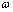= 0.9419 and maximum coefficient reliability which is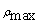= 0.9463. This coefficient reliability construction is categorized as a high coefficient, so that it can be said that the model is reliable.Download asVeiw figureFigures index
Figure 2. t-calculated of each indicator for every dimension in the First Trial Model

Because all fit model of loading factor value for each indicator in every dimension is more than 0.3; so that t-calculated value > 1.96; and its reliability construction is high. It can be stated that this model is good and there is no need for revision/modification. The same result is achieved from the second empirical trial model.

The trial (try out) hypothesis is done parametrically, because prerequisite test analysis for t-test (parametric) has fulfilled the assumptions of homogeneity and normality. t-paired test sample is applied. Based on the result of the calculation, it is known that t-calculated value is -27.5032. The value of t-table with 5% significance level and 14 sample on one sided test is t0.05,13 = 2,1604. Because t-calculated > t-table therefore H0 is rejected, which means that mean of coefficient reliability of McDonald method is lower than mean of maximum method coefficient reliability.

### 4. Discussion

The value of McDonalds’ coefficient reliability is lower than Maximum reliability coefficient because Maximum reliability coefficient formula has a variance error (θ), which may cause the value of coefficient reliability higher.

The formula of Mcdonald Reliability Coefficient  Is: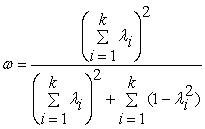The formula of Maximum coefficient reliability is: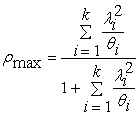λ = loading factor, θ = 1 – λ2 = variance error

The amount of coefficient reliability depends on value of λ (loading factor) and the measurement error. Loading Factor is a variance contribution of indicators on its latent construction. The higher the variance, the greater the coefficient reliability. Because Maximum coefficient reliability arranges the value of variance error, therefore the value tends to be higher than McDonald coefficient reliability. This can be proven mathematically as follows.

For the case of loading factor, k = 1 rate (only 1 component) or some other components which have same value, for example λ, both coefficients reliability value are same. It proves that the ratio(r) of McDonald and Maximum coefficient reliability moves from 0 up to 1. For loading factor k = 2 rate (2 different components), such as λ1 and λ2, the ratio is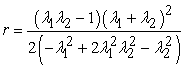. The visualization of r- equation can be seen in the following figure.Download asVeiw figureFigures index
Figure 3. Equation GraphicsFrom visualization of graph on figure 3 above, it can be clearly seen that r- value moves from 0 up to 1, with 0 ≤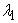≤ 1 and 0 ≤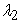≤ 1 domain, whereis represented by x andis represented by y. Maximum value of r is 1. It proves that McDonald coefficient reliability is lower than Maximum coefficient reliability ().

This is in line with a study done by Margono (2013). In his study, he estimated that multidimensional coefficient reliability on students’ attitude toward statistics’ measurement is consists of 3 dimensions. The finding of the study is the estimation of McDonald coefficient reliability which is 0.791 and maximum coefficient reliability which is 0.837.  It shows that McDonald coefficient reliability is lower than maximum reliability coefficient.

Generally, the quantitative and qualitative analytic result shows that non test performance device or affection instrument towards math for high school students which is developed based on theories, experts, and panelists’ review already developed empirically in some high schools. It is apropriate and can be applied for affection assessment in high school level. If it is compared to the concept and the previous draft from non test device, there are some revisions and developments which resulted from the rational try out by experts and panelists and also the empirical trials from respondents, similar for the first and second section. That development covers the compatibility between item and indicator, between indicator and dimension in its latent construction, and the using of more communicative language which can be understood by respondents.

Validity pertains with the extent in which a test is capable for measuring what supposed to be measured. Performance test which is developed has sufficient validity for both validity construction which has assessed by experts and panelists and also the validity construction from empirical try out. The validity construction is seen on the compatibility between item and its indicator. The indicator that form a dimension is a latent construction. This non test instrument is developed based on affection competences’ assessment device in high school level and theoritical review which supports that competence. The result of the experts’ assessment shows that this non test instrument has sufficient construct validity. Therefore, this non test instruments can be used for high school level, both state and private schools.

The reliability of this non test instrument is classified as a high level, whether if it was emphasized from the panelist assessments’ result, or from the first and second empirical try out. The construct coefficient reliability from the first and second empirical try out is more than 0.9. This coefficient reliability is classified as a high level, eventhough it closes to a perfect level. The measurement reliability of the device is consistence for the device to measure what to be measured. The higher coefficient reliability, the closer observed score to the real component score. The value of observed score can be used as a substitute of real component score. Therefore, it can be said that the result of the measurement, using this non test device, shows the participants’ competence which is close to their real competence.

However, target objects, e.g. students’ response, become a source of error which is needed to be evaluated in this measurement. The variety of students’ ability and comprehension toward questions in the research questionnaire influence the reliability of this non test device. Besides that, students’ psychological factor should be concerned when they are answering the questions. Students with a good mood will answer the questions well. On contrary, students with anxiousness, restless, or other psychological disturbance tend to answer the questions in desultory. Therefore, to guarantee having a high instrument reliability, the use of non test instrument at school must be conducted carefully and considered the psychological state of the students. In this case, teacher who gives this questionnaire needs to emphasize students’ readiness condition, whether the students are psychologically ready to answer the questions of non test instruments given.

### 5. Conclusion

Based on the result of the empirical try out which has been conducted, it shows that the instrument for measuring high school students’ affection towards math has fulfilled validity and reliability requirements. This can be proven by construct validity analysis using Maximum Likelihood method in confirmatory factor analysis. The calculation of construct reliability coefficient by using McDonald and Maximum method of multidimensional reliability formula shows a very high reliability coefficient. The result of try out (trial) hypothesis shows that McDonald reliability coefficient is lower than Maximum reliability coefficient. The comparison between McDonald reliability coefficient and Maximum reliability coefficient moves from 0 up to 1 loading factor. However, there has not been an agreement reached by experts of psychometric about the most reliable coefficient for a research instrument. It is expected that other reliability coefficients from classic test theory or modern test theory/ Item Response Theory (IRT) are used in future research.

### References

  Schunk, Dale H., Paul R. Pintrich, dan Judith L. Meece, Motivation in Education: Theory, Research, and Applications. Pearson Education, New Jersey Inc., 2008.In article  Smith, Mark K., Teori Pembelajaran dan Pengajaran, terjemahan Abdul Qodir Saleh. Mirza Media Pustaka, Yogyakarta, 2009.In article  Bloom, Benjamin S., Human Characteristics and School Learning. New York: McGraw-Hill Book Company, 1976.In article  Gronlund, Norman E., Measurement and Evaluation in Teaching. Macmillan Publishing Company, New York, 1985.In article  Clarkson, Philips dan Norma Presmeg, Critical Issues in Mathematics Education. Springer Science+Business Media, New York, 2008.In article  Widhiarso, Wahyu, “Koefisien Reliabilitas pada Pengukuran Kepribadian yang Bersifat Multidimensional.” Psikobuana, Vol 1 (1), 39-48. 2009In article  Widhiarso, Wahyu dan Djemari Mardapi, “Komparasi Ketepatan Estimasi Koefisien Reliabilitas Teori Skor Murni Klasik.” Unpublished, 2009.In article  Naga, Dali S., Teori Skor pada Pengukuran Mental. PT. Nagarani Citrayasa, Jakarta, 2013.In article  Margono, Gaguk, “Aplikasi Analisis Faktor Konfirmatori untuk Menentukan Reliabilitas Multidimensi.” Statistika, Vol. 13 (1), Mei, 17-24. 2013In article  McDonald, Roderick P., Test Theory: A Unified Treatment. New Jersey: Lawrence Erlbaum Associates, Inc., 1999.In article  Wahyu Widhiarso, “Estimasi Reliabilitas Pengukuran dalam Pendekatan Model Persamaan Struktural,” http://widhiarso.staff.ugm.ac.id. (accessed May 23, 2013).In article